Courses

# Test: Turing Machine: RE, REC & Undecidability- 2

## 15 Questions MCQ Test Question Bank for GATE Computer Science Engineering | Test: Turing Machine: RE, REC & Undecidability- 2

Description
This mock test of Test: Turing Machine: RE, REC & Undecidability- 2 for Computer Science Engineering (CSE) helps you for every Computer Science Engineering (CSE) entrance exam. This contains 15 Multiple Choice Questions for Computer Science Engineering (CSE) Test: Turing Machine: RE, REC & Undecidability- 2 (mcq) to study with solutions a complete question bank. The solved questions answers in this Test: Turing Machine: RE, REC & Undecidability- 2 quiz give you a good mix of easy questions and tough questions. Computer Science Engineering (CSE) students definitely take this Test: Turing Machine: RE, REC & Undecidability- 2 exercise for a better result in the exam. You can find other Test: Turing Machine: RE, REC & Undecidability- 2 extra questions, long questions & short questions for Computer Science Engineering (CSE) on EduRev as well by searching above.
QUESTION: 1

### Let M be a Turing Machine has Q = (q0, q1, q2, q3, q4} a set of states, input alphabets {0, 1}. The tape alphabets are {0,1, B, X, V). The symbol B is used to represent end of input string. The final state is q4. The transistions are as follows: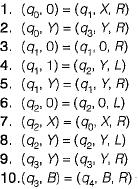Which of the following is true about M?

Solution:

Drawing the equivalent turing machine we have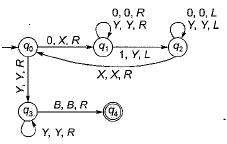This is a standard turing machine program for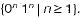QUESTION: 2

### If L1 and L2 are a pair of complementary languages. Which of the following statement is not possible?

Solution:

The correct statement follows from following theorem:
Theorem: If L1 and L2 is a pair of complementary language then either
• Both L1 and L2 are recursive.
• Neither L1, and L2 are recursively enumerable.
• One is recursively enumerable but not recursive, the other is not RE.
Option (b) is not possible, since if L1 is recursive, then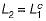will also be recursive.

QUESTION: 3

### Consider the following statements about L: (i) L is accepted by multi-tape turing machine M1. (ii) L is also accpted by a single tape turing machine M2. Which of the following statement is correct?

Solution:

While simulating multi-tape TM on a single tape TM, the head has to move at least 2k cells per move, where k is the number of tracks on single tape TM. Thus for k moves, we get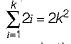which means quadratic slow down. Thus, acceptance by single-tape is slower by 0(n2).

QUESTION: 4

A TM M-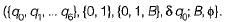The transition are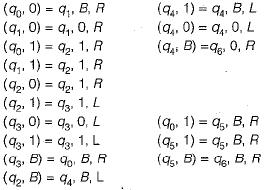What is the laugnage accepted by TM?

Solution:

The analysis of transition of TM, M shows that is accepts language 0*10*.

QUESTION: 5

Referring to Turing Machine in previous question, what will be the output when the input is
I1 = 0100 and I2 = 0010?

Solution:

The Turing Machine carries out the functions of ( m - n) in (0m 10n).
• When m≥n, the output is difference between m and n.
• when m < n, the output is blank.

QUESTION: 6

Nobody knows yets if p = NP consider the language L defined as follows: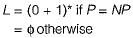Which of the following statement is true?

Solution:

L = (0 + 1 )* or {}. A trivial TM which accepts all words or another which rejects all. One of these two TMs will surely accept this language. So L is recursive.

QUESTION: 7

If the strings of a language L can be effectively enumerated in lexicographic (i.e. alphabetic) order which of the following statements is true?

Solution:

L is recursive iff L is enumerable in Lexicographic order (alphabetic).
It is also known that context free language is a subset of recursive languages.

QUESTION: 8

he diagnoalization language, Ld is a

Solution: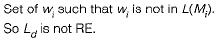QUESTION: 9

Time taken by one tape TM to simulate n moves of k-tape TM is

Solution:

Single tape turing machine can simulate n-moves of k-tape TM in O(n2).

QUESTION: 10

Consider the transition table of a TM given below. Here "b" represents the blank symbol.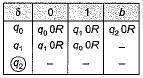Q. Which of the following strings will not accepted?

Solution: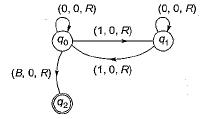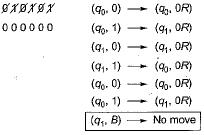Hence, not accepted.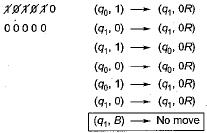Hence, also not accepted.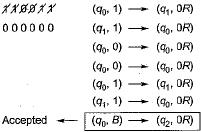QUESTION: 11

The given turing machine accepts

Solution:

It can be observed from the image that this machine will accept only those strings which contains even number of 1’s. And strings with odd number of 1 ’s are rejected.

QUESTION: 12

Ram and Shyam have been asked to show that a cartain problem II is NP-complete. Ram shows a polynomial time reduction from the 3-SAT problem to II and Shyam shows a polynomial time reduction from II to 3-SAT. Which of the following can be inferred from these reductions?

Solution:

Since 3-SAT problem is NP-complete and 3-SAT is polynomial time reducible to π hence π is is also NP-complete.

QUESTION: 13

Which of the following is true?

Solution: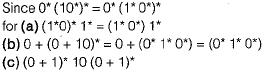QUESTION: 14

Both P and NP are closed under the operation of

Solution:

P and NP language are closed under union, intersection, kleene closure and concatenation, P is close under complement also while closure of NP under complement is still unsolved problem.

QUESTION: 15

L ∈ NP does not obviously imply that

Solution:

L ∈ NP does not imply that L' ∈ NP. Since closure of NP under complementation is as yet unresolved problem. Also L ∈ NP does not imply L' ∈ P, unless L ∈ P, which may or may not be so.